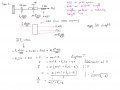# "Simple" Free Body Diagram( Control Systems Engineering) How to apply forces

#### EB(E/A)E

Joined Mar 29, 2020
24
The problem is as follows to write an equation for this system. However, by following guides on Youtube I get my answer as shown in my embedded picture. Tho the real answer is something else:
X(s)/F(s) = 1/ 5s(s+0.8)

I didnt include the values of the constants my attempts, I just want to get the expression correct. I would assume my error is in how I apply the forces on the FBD(Free Body Diagram).#### drc_567

Joined Dec 29, 2008
1,156
Try using $$F=-kX$$.

•EB(E/A)E